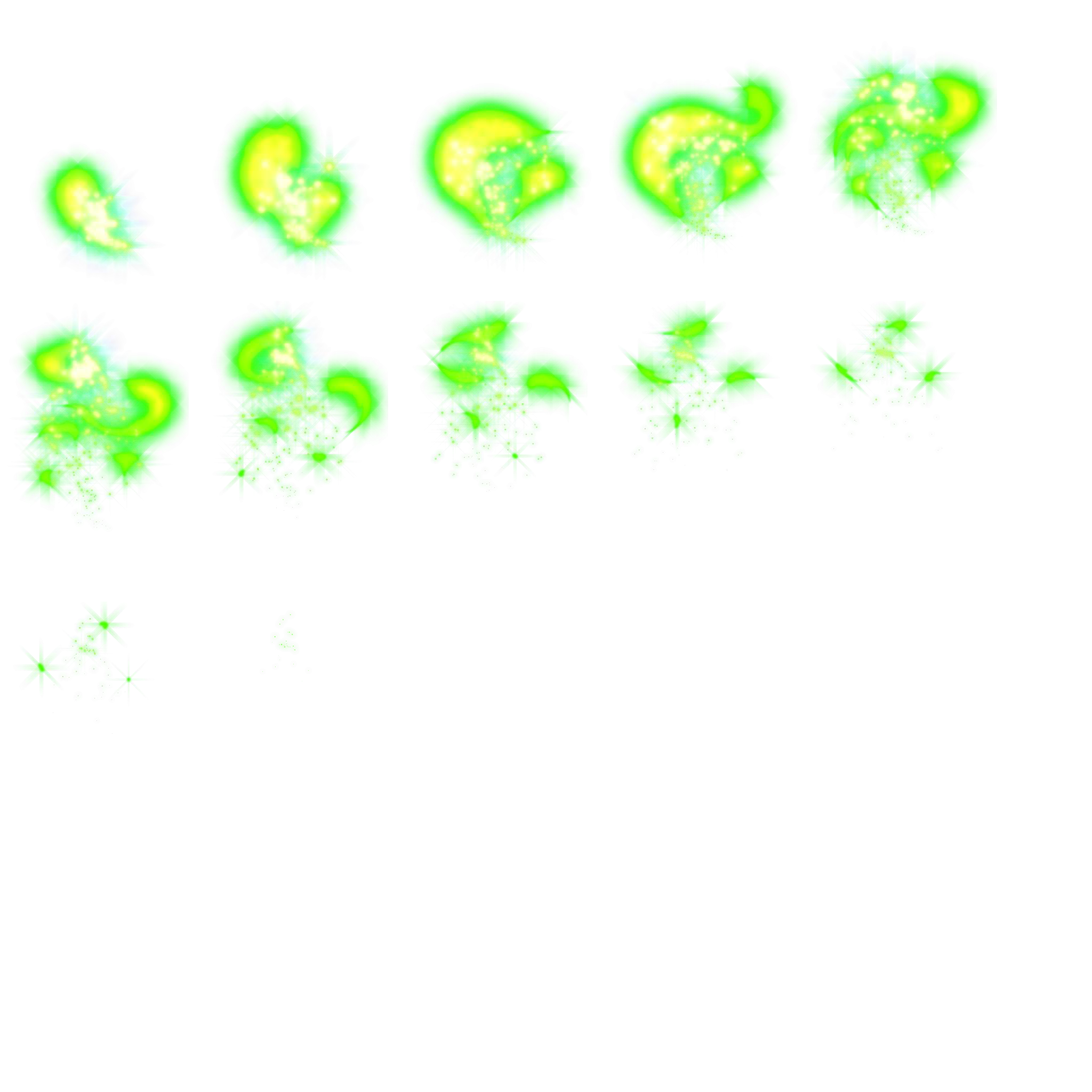i

## Missing Divisor# Missing Divisor

Improve your students’ math facts with this magical missing number game.  By selecting the missing divisor to complete the equation, kids practice their math skills and build their algebraic thinking. Multiplication and division won’t be so tricky after solving these math equations.

### Common Core

Grade 3 » Operations & Algebraic Thinking

CCSS.MATH.CONTENT.3.OA.A.4
Determine the unknown whole number in a multiplication or division equation relating three whole numbers. For example, determine the unknown number that makes the equation true in each of the equations 8 × ? = 48, 5 = _ ÷ 3, 6 × 6 = ?

i